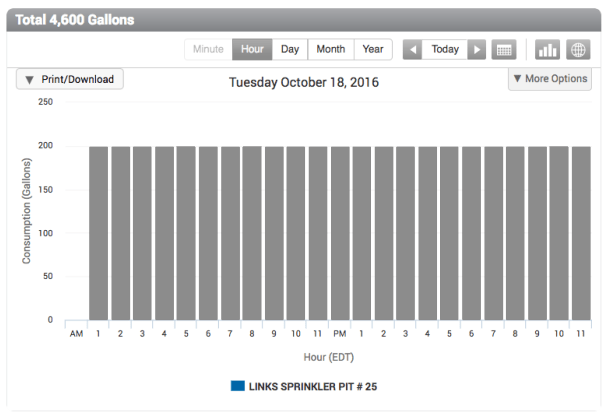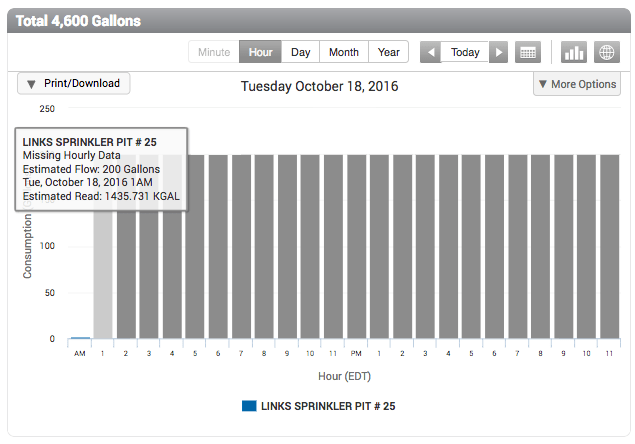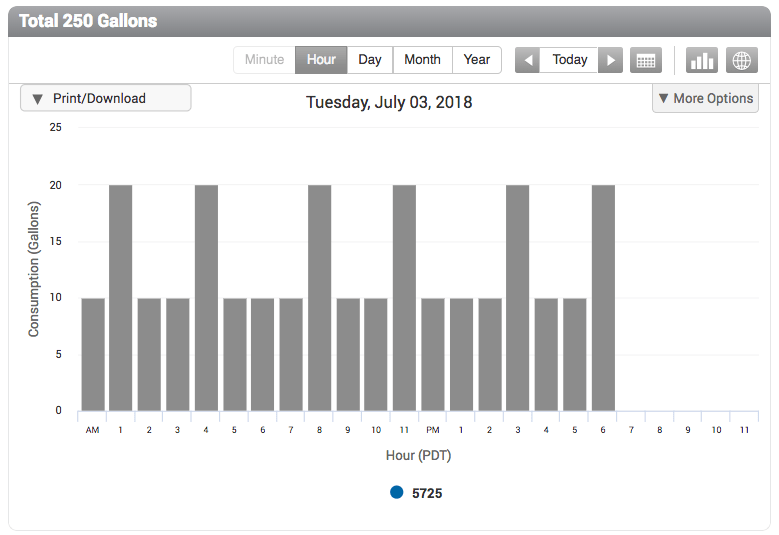# Endpoints, Data Backfill and Estimated Reads

Q: What happens when communications between an endpoint and AquaCUE is interrupted? Will missing reads be backfilled when communication is re-established? When does AquaCUE estimate reads?
A:
“Backfill” is the process of filling in missing data, typically meter reads that were not received because a scheduled communication with an endpoint was missed. If an endpoint has not communicated with AquaCUE for more than 24 hours, when communication is re-established and the next endpoint message includes the missing reads, those reads will be “backfilled” and shown in the Monitor page Consumption Graph. If the next endpoint message does not include the missing reads, AquaCUE estimates reads over the time period by calculating the total amount of consumption that occurred and evenly distributing it across the gap. This produces Consumption Graphs that look like the one below.Gray bars tell when consumption data is an estimate. To get more details:

1. Hover the cursor over a gray bar in the Monitor page consumption graph. The tooltip shows the total estimated flow that was calculated as an average from missing hourly data.2. Use the Actions drop-down menu to, for example, Export Data>Date Range and choose a start date that comes before the date displayed in the Consumption Graph. Then open the file in Excel to see if an estimated flag was set by looking at the Estimated Flag column (column AV in the example below).

In addition you can look at columns:

• L to see when flow was reported by the endpoint
• N to see the flow total.AquaCUE calculated the total flow measured between the valid read of 1435.531 at 0:59 on the 18th and the valid read of 1442.337 at 11:59 on the 19th. It then evenly distributed the result of 6.806 KGallons (the unit of measure in this example) as estimated reads across the 34 hours between valid reads.The data in this example is the same data that generated the Consumption Graph for LINKS SPRINKLER PIT #25 (above).

• There are times when estimated reads get distributed unevenly, as shown in the Consumption Graph below.This uneven distribution is the result of meter resolution, which in this example is 10. That is, each revolution of the meter’s disc measures 10 gallons. As a result, AquaCUE distributes estimated reads for this particular meter in 10-gallon increments. Between 12 AM and 6 PM, a total of 250 gallons was used, which AquaCUE distributed as 10 + 20 + 10 + 10 + 20 + 10 + 10 + 10 + 20 + 10 + 10 + 20 + 10 + 10 + 10 + 20+ 10 + 10 + 20 = 250.